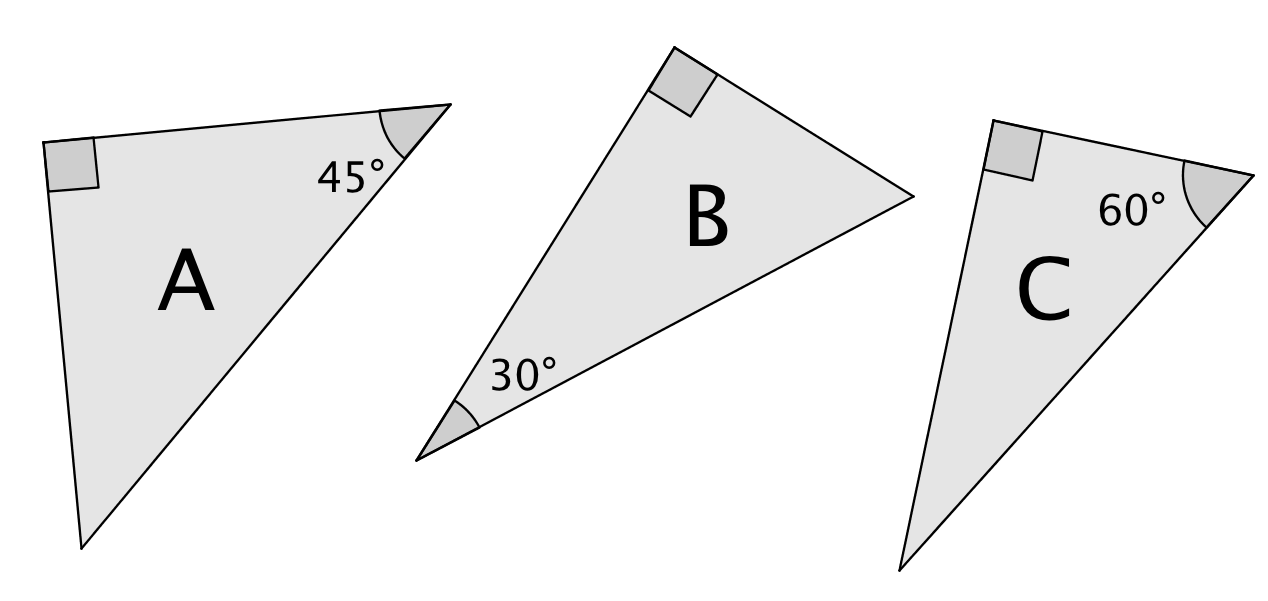# Right Triangles and Trigonometry

Lesson 8

Math

Unit 4

Lesson 8 of 19

## Objective

Derive the relationship between sine and cosine of complementary angles in right triangles, and describe sine and cosine as angle measures approach 0°, 30°, 45°, 60°, and 90°.

## Common Core Standards

### Core Standards

• G.SRT.C.7 — Explain and use the relationship between the sine and cosine of complementary angles.

• G.CO.C.10

## Criteria for Success

1. Describe that the value of sine approaches 1 and the value of the cosine approaches 0 as an angle measure approaches 90°.
2. Describe that the value of sine approaches 0 and the value of the cosine approaches 1 as an angle measure approaches 0°.
3. Derive the relationship between sine and cosine of complementary angles in right triangles using the reference angles of 30°/60°, 45°/45°, 90°/0°.
4. Extend the relationship of sine and cosine of complementary angles to non-reference angles in right triangles. ​​​​​​
Fishtank Plus

Unlock features to optimize your prep time, plan engaging lessons, and monitor student progress.

## Anchor Problems

### Problem 1

Below are three right triangles. Assume the value of the hypotenuse of each triangle is $$1$$.a) Using what you know about special right triangles, find the length of each side.
b) Fill in the chart describing the sine and cosine of each measure below.

 $${\mathrm{sin}(30^\circ)}$$ $${\mathrm{sin}(45^\circ)}$$ $${\mathrm{sin}(60^\circ)}$$ $${\mathrm{cos}(30^\circ)}$$ $${\mathrm{cos}(45^\circ)}$$ $${\mathrm{cos}(60^\circ)}$$

### Problem 2

Using the cosine and sine values from the table in Anchor Problem #1, identify trigonometric ratios that are the same. Then, write a conjecture about how the sine is related to the cosine of complementary angles.

## Problem Set

The following resources include problems and activities aligned to the objective of the lesson that can be used to create your own problem set.

FInd the value of $${\theta}$$ that makes each statement true.

$$\mathrm{sin}{\theta}=\mathrm{cos}32$$

$$\mathrm{cos}{\theta}=\mathrm{sin}({\theta}+20)$$

#### References

EngageNY Mathematics Geometry > Module 2 > Topic E > Lesson 27Exit Ticket, Question #1

Geometry > Module 2 > Topic E > Lesson 27 of the New York State Common Core Mathematics Curriculum from EngageNY and Great Minds. © 2015 Great Minds. Licensed by EngageNY of the New York State Education Department under the CC BY-NC-SA 3.0 US license. Accessed Dec. 2, 2016, 5:15 p.m..

Lesson 7

Lesson 9

## Lesson Map

Topic A: Right Triangle Properties and Side-Length Relationships

Topic B: Right Triangle Trigonometry

Topic C: Applications of Right Triangle Trigonometry

Topic D: The Unit Circle

Topic E: Trigonometric Ratios in Non-Right Triangles